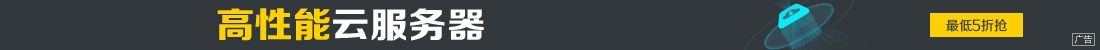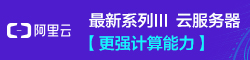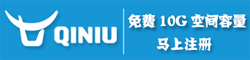# thinkPHP中volist标签用法示例

••name（必须） 要输出的数据模板变量 id（必须） 循环变量 offset（可选） 要输出数据的offset length（可选） 输出数据的长度 key（可选） 循环的key变量，默认值为i mod（可选） 对key值取模，默认为2 empty（可选） 如果数据为空显示的字符串

```\$User = M('User');
\$list = \$User->select();
\$this->assign('list',\$list);

```
```<volist name="list" id="vo">
{\$vo.id}
{\$vo.name}
</volist>

```

```<volist name="list" id="vo" mod="2" >
<eq name="mod" value="1">{\$vo.name}</eq>
</volist>

```

mod从0开始计数，这里mod循环计数为0,1

Mod属性还用于控制一定记录的换行，例如：

```<volist name="list" id="vo" mod="5" >
{\$vo.name}
<eq name="mod" value="4"><br/></eq>
</volist>

```

mod循环计数，0,1,2,3,4 当值为4的时候换行。也就是5条数据换一行。

```<volist name="list" id="vo" key="k" >
{\$k}.{\$vo.name}
</volist>

```

```<volist name="list" id="vo" >
{\$i}.{\$vo.name}
</volist>

```

```<volist name="agentData" id="vo" mod="3" key="k" empty="暂时没有数据">
<div style="display:inline-block;width:280px;margin:3px;">
</div>
{sh:\$mod}
<eq name="mod" value="2"><br/></eq>
</volist>

```